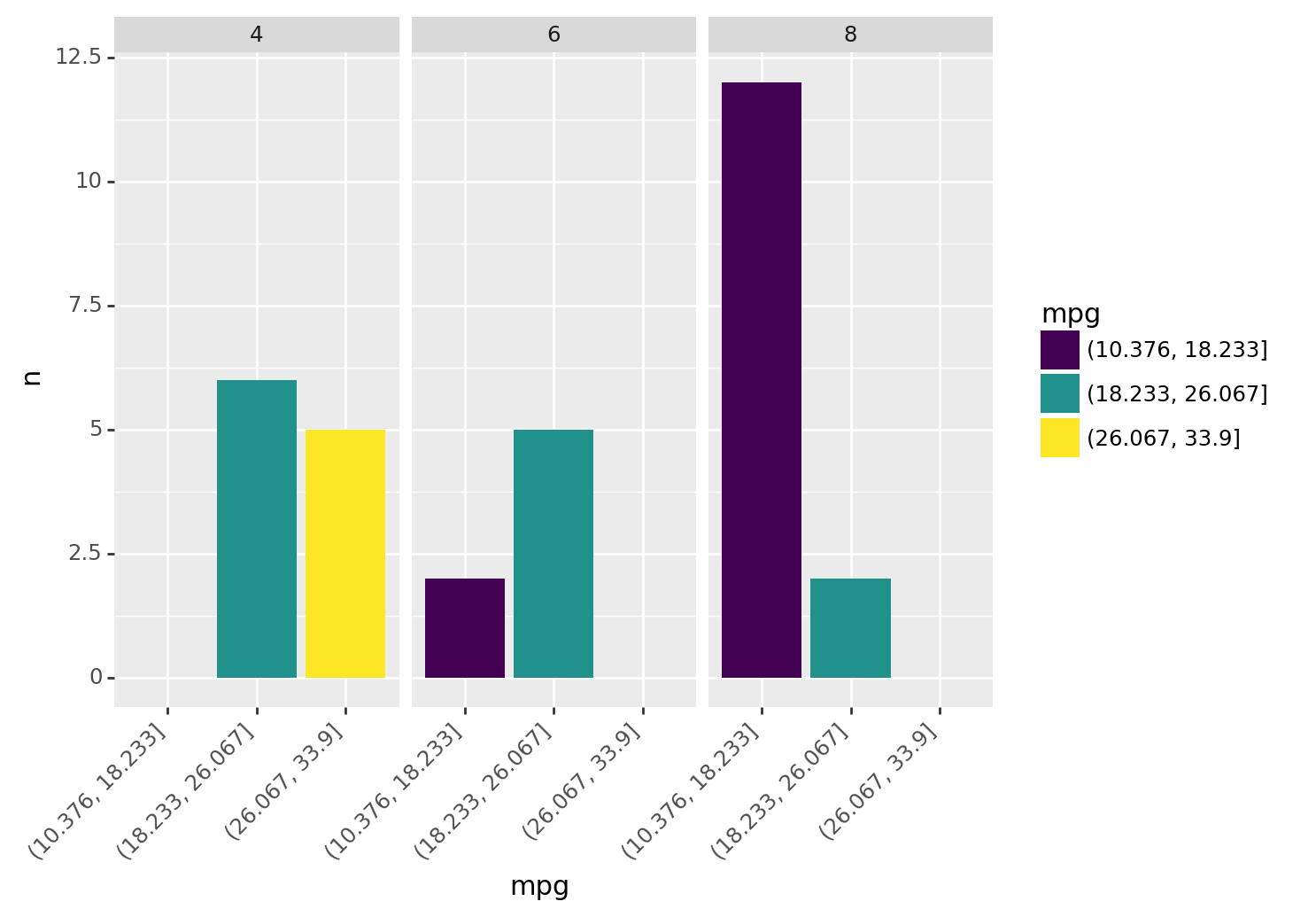Some combinations of verbs and column operations get used so frequently that they earn their own helper verbs. These helpers make things a little quicker or concise to type.

• `count()`: tally up values in the data.
• `separate()`: split a string column into separate columns (e.g. “a-1” -> “a” and “1”).
• `complete()`: expand and enhance data with all combinations of specific columns.
``````import pandas as pd
from siuba import _, count, separate, complete

from siuba.data import mtcars, penguins``````

## Count values

Use `count()` to quickly tally up the number of rows per grouping.

``mtcars >> count(_.cyl, _.gear)``
cyl gear n
0 4 3 1
1 4 4 8
... ... ... ...
6 8 3 12
7 8 5 2

8 rows × 3 columns

The output above has 8 rows—one for each grouping—and a column named `n` with the number of observations in each grouping.

Note that `count()` is a shorthand for combining `group_by()` and `summarize()`.

``````from siuba import group_by, summarize

mtcars >> group_by(_.cyl, _.gear) >> summarize(n = _.shape)``````
cyl gear n
0 4 3 1
1 4 4 8
... ... ... ...
6 8 3 12
7 8 5 2

8 rows × 3 columns

### Sorting results

Use the `sort` argument to sort results by number of observations, in descending order.

``mtcars >> count(_.cyl, sort=True)``
cyl n
0 8 14
1 4 11
2 6 7

### With expressions

``mtcars >> count(_.cyl, high_mpg = _.mpg > 30)``
cyl high_mpg n
0 4 False 7
1 4 True 4
2 6 False 7
3 8 False 14

Here’s a somewhat advanced example that puts mpg in different bins.

``````from siuba import count
from siuba.siu import call
from siuba.data import mtcars

car_counts = mtcars >> count(_.cyl, mpg = call(pd.cut, _.mpg, bins = 3))
car_counts``````
cyl mpg n
0 4 (10.376, 18.233] 0
1 4 (18.233, 26.067] 6
... ... ... ...
7 8 (18.233, 26.067] 2
8 8 (26.067, 33.9] 0

9 rows × 3 columns

Note these important pieces:

• `call(pd.cut, ...)` is used to lazily call `pd.cut(...)`.
• `pd.cut` splits the data into bins, that count then uses as a grouping.

Here’s a barchart of this data in plotnine.

``````from plotnine import ggplot, aes, geom_col, facet_wrap, theme, element_text

(car_counts
>> ggplot(aes("mpg", "n", fill="mpg"))
+ geom_col()
+ facet_wrap("~cyl")
+ theme(axis_text_x = element_text(angle=45, hjust=1))
)````````<ggplot: (8781310733834)>``

As vehicles increase in cylinders, they have fewer low mpg vehicles. For example, no `8` cylinder vehicles are in the highest `mpg` bin (above 26 mpg).

### Weighted counts

Use the `wt` argument to do a weighted count (i.e. sum the weight values per grouping).

This is useful if you have data that already contains a count, such as the count of `cyl` and `gear` below.

``tbl_n_cyl_gear = mtcars >> count(_.cyl, _.gear)``

The `wt` argument lets us roll this result up to count observations per `cyl`.

``tbl_n_cyl_gear >> count(_.cyl, wt=_.n)``
cyl nn
0 4 11
1 6 7
2 8 14

Notice that this is equivalent to counting `cyl` on the raw data.

``mtcars >> count(_.cyl)``
cyl n
0 4 11
1 6 7
2 8 14

## Separate strings into columns

Use `separate()` to split a column using a pattern, and produce new columns.

By default, it splits strings on any non-alphanumeric character, so is helpful for quickly splitting in common situations where values are seperated by dashes (e.g. “a-1”).

For example, here is some data where the `condition` column could be split on `"-"`.

``````measures = pd.DataFrame({
"condition": ["a-1", "a-2", "b-1", "b-2"],
"value": [1, 2, 3, 4]
})

measures``````
condition value
0 a-1 1
1 a-2 2
2 b-1 3
3 b-2 4

Separate takes the column we want to split, the names of the new columns. It produces a new table with the new columns in the place of the old one.

``measures >> separate(_.condition, ["name", "number"])``
value name number
0 1 a 1
1 2 a 2
2 3 b 1
3 4 b 2

Notice that the `condition` column got split into `name` and `number` columns.

## Complete combinations of data

Use `complete()` to fill in missing combinations in the data.

For example, the code below counts penguins across `species` and `island`, but not all species are on each island.

``````tbl_n_penguins = penguins >> count(_.species, _.island)
tbl_n_penguins``````
species island n
3 Chinstrap Dream 68
4 Gentoo Biscoe 124

Notice the following in the count:

• Adelie penguins are on three islands, BUT
• Chinstrap are only on Dream.
• Gentoo are only on Biscoe.

We can use complete to add rows with 0 counts for islands Chinstrap and Gentoo aren’t on.

``tbl_n_penguins >> complete(_.species, _.island, fill = {"n": 0})``
species island n
Now the data has 9 rows, one for each combination of species and island. The `fill = {"n": 0}` argument allowed us to set a default value of 0 for the previously missing rows.